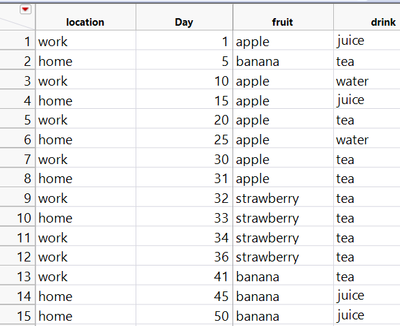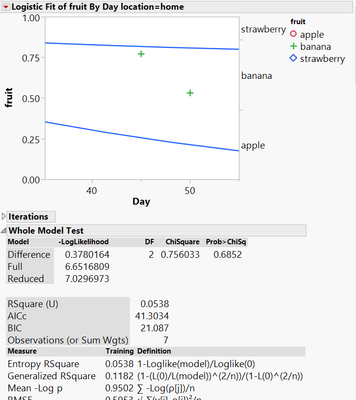Choose Language Hide Translation Bar
Highlighted

## How to remove summary statistics using JSL Part 3

Hello Community,

I had asked a related question to this yesterday and got great answers. However, I ran into problems when trying to implement the solution. After spending countless hours, I am asking for more help.

Here is the scenario.

I have a data table (attached) with the following columns and rows:1. I would like to loop through each column at a time starting from column "fruit" and plot a Logistic plot by "location" and send the output to a journal.

2. I would like to completely remove the summary statistics which come with the plots using JSL.

I can do step 1 with no issues. Below is my code:

``````dt=open("\$\fruit_drink.jmp");

colList = dt << Get Column Names();

For( i = 3, i <= Nitems( colList ), i++,
obj = Logistic(
Y( colList[i] ),
X( :Day ),
By( :location),
SendToReport(
Dispatch( {}, "1", ScaleBox, {Min( 35.2151394422311 )} ),
Dispatch(
{},
"FitNom Plot",
FrameBox,
{Marker Size( 4 ), Row Legend(
colList[i],
Color( 1 ),
Color Theme( "JMP Default" ),
Marker( 1 ),
Marker Theme( "Standard" ),
Continuous Scale( 0 ),
Reverse Scale( 0 ),
Excluded Rows( 0 )
)}
)
)
);
obj << journal;
);``````

I get this output (there are more graphs with summary statistics, I just can't fit all of them here):I tried to implement the following solution to remove the statistics (based on previous help I received from the JMP community).

``````dt=open("\$\fruit_drink.jmp");

colList = dt << Get Column Names();

For( i = 3, i <= Nitems( colList ), i++,
obj = Logistic(
Y( colList[i] ),
X( :Day ),
By( :location),
SendToReport(
Dispatch( {}, "1", ScaleBox, {Min( 35.2151394422311 )} ),
Dispatch(
{},
"FitNom Plot",
FrameBox,
{Marker Size( 4 ), Row Legend(
colList[i],
Color( 1 ),
Color Theme( "JMP Default" ),
Marker( 1 ),
Marker Theme( "Standard" ),
Continuous Scale( 0 ),
Reverse Scale( 0 ),
Excluded Rows( 0 )
)}
)
)
);
obj << journal;
jrn = New Window( "The Journal", <<journal );
For( i = 1, i <= N Items( obj ), i++,
obj[i] << journal;);
obj << close window;
For( i = 1, i <= N Items( obj ), i++,
jrn["Iterations"] << delete;
jrn["Whole Model Test"] << delete;
jrn["Parameter Estimates"] << delete;);

);

``````

When I run this script, I get an infinite loop and had to terminate JMP. When I put the inner for loops on the outside, nothing happens, the summary statistics do not get removed.

Can someone help me?

Thanks.

1 ACCEPTED SOLUTION

Accepted Solutions
Highlighted

## Re: How to remove summary statistics using JSL Part 3

simple solution.  The second For() loop in the script is located within the 1st For() loop, and they were both using "i" as the index variable.  So the second loop was continually setting the value of i back to a value less than the stopping value in the outside(first) loop.

Here is the simple fix

``````Names Default To Here( 1 );
dt = data table( "fruit_drink" );

colList = dt << Get Column Names();

jrn = New Window( "The Journal", <<journal );

For( i = 3, i <= N Items( colList ), i++,
obj = Logistic(
Y( colList[i] ),
X( :Day ),
By( :location ),
SendToReport(
Dispatch( {}, "1", ScaleBox, {Min( 35.2151394422311 )} ),
Dispatch(
{},
"FitNom Plot",
FrameBox,
{Marker Size( 4 ), Row Legend(
colList[i],
Color( 1 ),
Color Theme( "JMP Default" ),
Marker( 1 ),
Marker Theme( "Standard" ),
Continuous Scale( 0 ),
Reverse Scale( 0 ),
Excluded Rows( 0 )
)}
)
)
);
obj << journal;

For( k = 1,k <= N Items( obj ), k++,
Try( jrn["Iterations"] << delete );
Try( jrn["Whole Model Test"] << delete );
Try( jrn["Parameter Estimates"] << delete );
);

Try( obj << close window );

);``````
Jim
6 REPLIES 6
Highlighted

## Re: How to remove summary statistics using JSL Part 3

There are a few issues with your code

1. You should really be adding as the first line in your code
``Names Default To Here( 1 );``
it keeps your variables in this script from interfering with same named variables in other script
2. You are attempting to use objects and variables before they are created or after they have been deleted
``````obj << journal;
jrn = New Window( "The Journal", <<journal );``````
The above case is an illustration of attempting to send something to a journal, before you create the journal
``````obj << close window;
For( i = 1, i <= N Items( obj ), i++,
jrn["Iterations"] << delete;
jrn["Whole Model Test"] << delete;
jrn["Parameter Estimates"] << delete;);

);``````
And in this case, you are referencing an object that you have just deleted
3. I discovered that in some cases, there isn't an Iterations table, and the code would error out, so I have added a Try() function around some of the statements.
``````For( i = 1, i <= N Items( obj ), i++,
Try( jrn["Iterations"] << delete );
Try( jrn["Whole Model Test"] << delete );
Try( jrn["Parameter Estimates"] << delete );
);

Try( obj << close window );``````
The Try() function does what it says, it just "Tries" to do the statement, but if it can't do it, it just keeps going.
4. You also seemed to attempt a second way of adding your code to the journal.
``````obj << journal;
jrn = New Window( "The Journal", <<journal );
For( i = 1, i <= N Items( obj ), i++,
obj[i] << journal;);``````
I assume it is not required, and is only there because of the issue of attempting to upload to the journal before it was created.

So here is a rework of your code.

``````Names Default To Here( 1 );
dt = Open( "\$\fruit_drink.jmp" );

colList = dt << Get Column Names();

jrn = New Window( "The Journal", <<journal );

For( i = 3, i <= N Items( colList ), i++,
obj = Logistic(
Y( colList[i] ),
X( :Day ),
By( :location ),
SendToReport(
Dispatch( {}, "1", ScaleBox, {Min( 35.2151394422311 )} ),
Dispatch(
{},
"FitNom Plot",
FrameBox,
{Marker Size( 4 ), Row Legend(
colList[i],
Color( 1 ),
Color Theme( "JMP Default" ),
Marker( 1 ),
Marker Theme( "Standard" ),
Continuous Scale( 0 ),
Reverse Scale( 0 ),
Excluded Rows( 0 )
)}
)
)
);
obj << journal;

For( i = 1, i <= N Items( obj ), i++,
Try( jrn["Iterations"] << delete );
Try( jrn["Whole Model Test"] << delete );
Try( jrn["Parameter Estimates"] << delete );
);

Try( obj << close window );

);``````
Jim
Highlighted

## Re: How to remove summary statistics using JSL Part 3

Hi txnelson,

Thanks for taking your time to help me out. It looks like your solution is close to what I want. The only issue is that when running the script, JMP goes in an infinite loop. It keeps appending charts to the journal without stopping forcing me to kill the session.

Thanks.

Highlighted

## Re: How to remove summary statistics using JSL Part 3

It did not do that in my testing of the code I submitted.  Can you post your data table.  I assume that the issue is a data issue, and if I had a copy of your data table I could look further.

Jim
Highlighted

## Re: How to remove summary statistics using JSL Part 3

Hi txnelson,

Attached is my data table. That is strange that it runs fine for you.

Thanks.

Highlighted

## Re: How to remove summary statistics using JSL Part 3

simple solution.  The second For() loop in the script is located within the 1st For() loop, and they were both using "i" as the index variable.  So the second loop was continually setting the value of i back to a value less than the stopping value in the outside(first) loop.

Here is the simple fix

``````Names Default To Here( 1 );
dt = data table( "fruit_drink" );

colList = dt << Get Column Names();

jrn = New Window( "The Journal", <<journal );

For( i = 3, i <= N Items( colList ), i++,
obj = Logistic(
Y( colList[i] ),
X( :Day ),
By( :location ),
SendToReport(
Dispatch( {}, "1", ScaleBox, {Min( 35.2151394422311 )} ),
Dispatch(
{},
"FitNom Plot",
FrameBox,
{Marker Size( 4 ), Row Legend(
colList[i],
Color( 1 ),
Color Theme( "JMP Default" ),
Marker( 1 ),
Marker Theme( "Standard" ),
Continuous Scale( 0 ),
Reverse Scale( 0 ),
Excluded Rows( 0 )
)}
)
)
);
obj << journal;

For( k = 1,k <= N Items( obj ), k++,
Try( jrn["Iterations"] << delete );
Try( jrn["Whole Model Test"] << delete );
Try( jrn["Parameter Estimates"] << delete );
);

Try( obj << close window );

);``````
Jim
Highlighted

## Re: How to remove summary statistics using JSL Part 3

Beautiful solution. That is what I needed. Thanks txnelson for your quick response. Much appreciated!
Article Labels